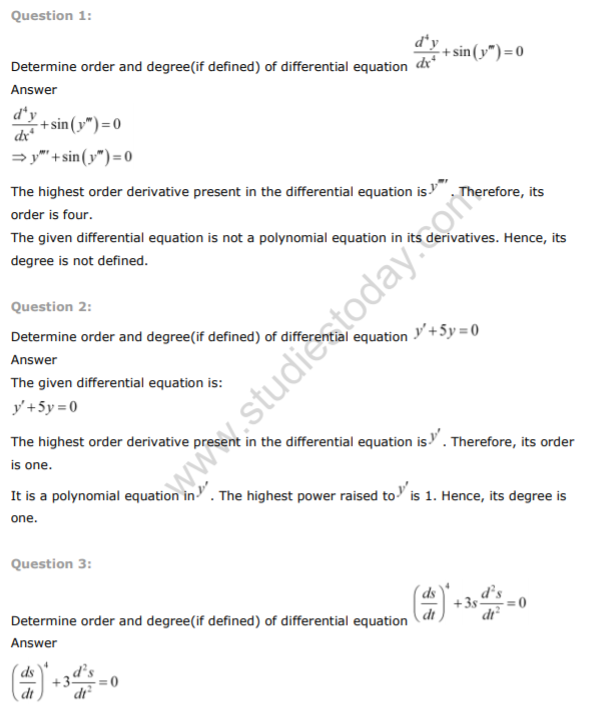# NCERT Class 12 Maths Solutions Differential Equations

## Differential Equations Class 12 NCERT Solutions

Class 12 Mathematics students should refer to the following NCERT questions with answers for Differential Equations in standard 12. These NCERT Solutions with answers for Grade 12 Mathematics will come in exams and help you to score good marks

### Differential Equations NCERT Solutions Class 12

MATHSNCERT Class 12 Maths Solutions Relations and Functions
 NCERT Class 12 Maths Solutions Vector Algebra
 NCERT Class 12 Maths Solutions Three Dimensional Geometry
 NCERT Class 12 Maths Solutions Linear Programming
 NCERT Class 12 Maths Solutions Probability
 NCERT Class 12 Maths Solutions Inverse Trigonometric Functions
 NCERT Class 12 Maths Solutions Matrices
 NCERT Class 12 Maths Solutions Determinants
 NCERT Class 12 Maths Solutions Continuity and Differentiability
 NCERT Class 12 Maths Solutions Application of Derivatives
 NCERT Class 12 Maths Solutions Integrals
 NCERT Class 12 Maths Solutions Application of Integrals
 NCERT Class 12 Maths Solutions Differential Equations

Tags